# Properties of a Parallelogram

In Geometry, a parallelogram is a type of quadrilateral. It is a two-dimensional figure with four sides. The most important properties of a parallelogram are that the opposite sides are parallel and congruent and the opposite angles are also equal. In this article, let us discuss all the properties of a parallelogram with a complete explanation and many solved examples.

## Important Properties of a Parallelogram

A parallelogram is a closed four-sided two-dimensional figure in which the opposite sides are parallel and equal in length. Also, the opposite angles are also equal. Learning the properties of a parallelogram is useful in finding the angles and sides of a parallelogram. The four most important properties of a parallelogram are:

• The opposite sides of a parallelogram are equal in measurement and they are parallel to each other.
• The opposite angles of a parallelogram are equal.
• The sum of interior angles of a parallelogram is equal to 360°.
• The consecutive angles of a parallelogram should be supplementary (180°).

## Theorems on Properties of a Parallelogram

The 7 important theorems on properties of a parallelogram are given below:

### Theorem 1:

A diagonal of a parallelogram divides the parallelogram into two congruent triangles.

Proof: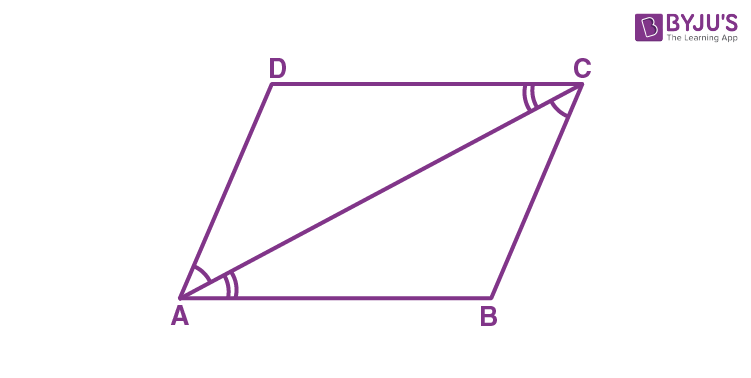Assume that ABCD is a parallelogram and AC is a diagonal of a parallelogram.

The diagonal AC divides the parallelogram into two congruent triangles, such as ∆ABC and ∆CDA. Now, we need to prove that the triangles ∆ABC and ∆CDA are congruent.

From the triangles, ∆ABC and ∆CDA, AD || BC, and AC is a transversal.

Hence, ∠ BCA = ∠ DAC (By using the property of pair of alternate angles)

Also, we can say that AB || DC and line AC is transversal.

Thus, ∠ BAC = ∠ DCA (Using the pair of alternate angles)

Also, AC = CA (Common side)

By using the Angle side Angle rule (ASA rule), we can conclude that ∆ABC is congruent to ∆CDA.

(i.e) ∆ABC ≅ ∆CDA.

Thus, the diagonal AC divides a parallelogram ABCD into two congruent triangles ABC and CDA.

Hence, proved.

### Theorem 2:

The opposite sides of a parallelogram are equal.

Proof:

From theorem 1, it is proved that the diagonals of a parallelogram divide it into two congruent triangles. When you measure the opposite sides of a parallelogram, it is observed that the opposite sides are equal.

Hence, we conclude that the sides AB = DC and AD = BC.

### Theorem 3:

If each pair of opposite sides of a quadrilateral is equal, then the quadrilateral is a parallelogram.

Proof: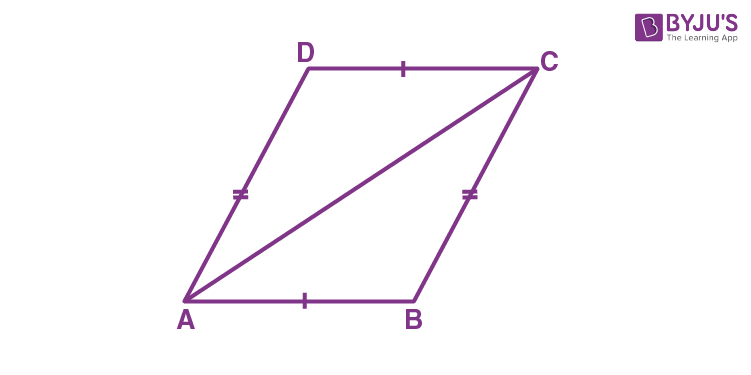Assume that the sides AB and CD of the quadrilateral ABCD are equal and also AD = BC

Now, draw the diagonal AC.

Clearly, we can say that ∆ ABC ≅ ∆ CDA (From theorem 1)

Therefore, ∠ BAC = ∠ DCA and ∠ BCA = ∠ DAC.

From this result, we can say that the quadrilateral ABCD is a parallelogram because each pair of opposite sides is equal in measurement.

Thus, conversely, we can say that if each pair of opposite sides of a quadrilateral is equal, then the quadrilateral is a parallelogram.

Hence, proved.

### Theorem 4:

The opposite angles are equal in a parallelogram.

Proof:

Using Theorem 3, we can conclude that the pairs of opposite angles are equal.

(i.e) ∠A = ∠C and ∠B = ∠D

Thus, each pair of opposite angles is equal, a quadrilateral is a parallelogram.

### Theorem 5:

If in a quadrilateral, each pair of opposite angles is equal, then it is a parallelogram.

Proof:

We can say that Theorem 5 is the converse of Theorem 4.

### Theorem 6:

The diagonals of a parallelogram bisect each other.

Proof:

Consider a parallelogram ABCD and draw both the diagonals and it intersects at the point O.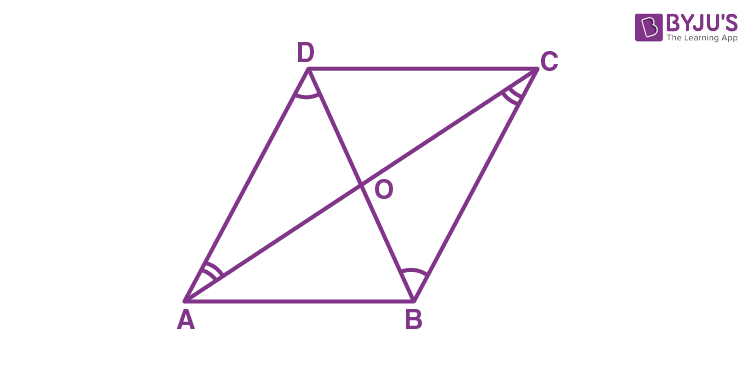Now, measure the lengths, such as OA, OB, OC and OD.

You will observe that OA = OC and OB = OD

So, we can say that “O” is the midpoint of both the diagonals.

Hence, proved.

### Theorem 7:

If the diagonals of a quadrilateral bisect each other, then it is a parallelogram.

Proof:

This theorem is the converse of Theorem 6.

Now, consider a parallelogram ABCD which is given below: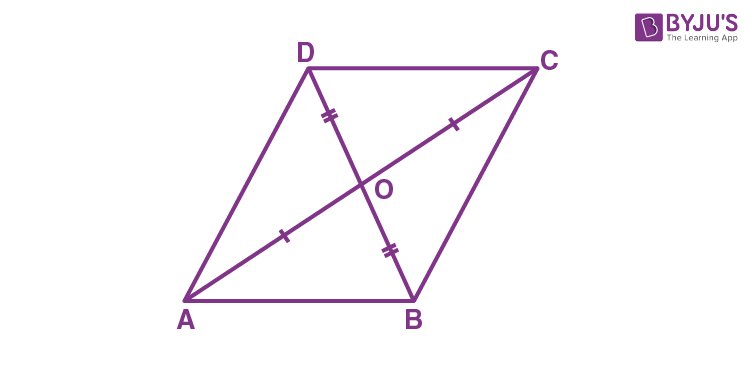From the figure, we can observe that OA = OC and OB = OD.

Hence, we can say ∆ AOB ≅ ∆ COD.

∠ ABO = ∠ CDO

Thus, we can conclude that AB || CD and BC || AD.

Hence, ABCD is a parallelogram.

### Properties of a Parallelogram Example

Question:

Prove that the bisectors of angles of a parallelogram form a rectangle.

Solution:

Assume that ABCD is a parallelogram. Let P, Q, R, S be the point of intersection of bisectors of

∠A and ∠B, ∠B and ∠C, ∠C and ∠D, and ∠D and ∠A, respectively.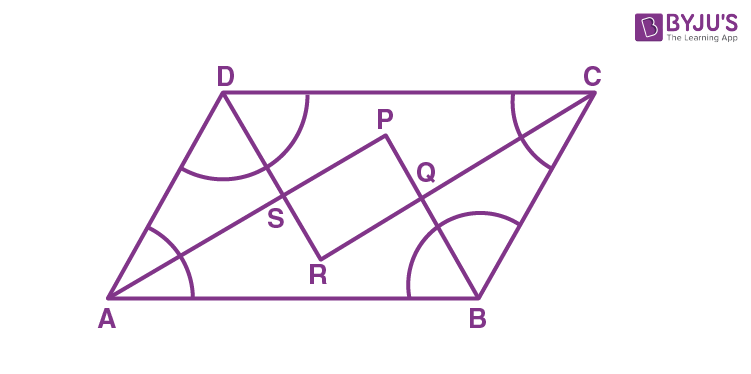From the triangle, ASD, we can observe that DS bisects ∠D and AS bisects ∠A.

Therefore,

∠ADS + ∠DAS = (½)∠D + (½)∠A

∠ADS + ∠DAS = ½ (∠A + ∠D)

∠ADS + ∠DAS = ½ (180°) [Since ∠A and ∠D are the interior angles on the same side of the transversal]

Therefore, ∠ADS + ∠DAS = 90°.

Using the angle sum property of a triangle, we can write:

∠ DAS + ∠ ADS + ∠ DSA = 180°

Now, substitute ∠ADS + ∠DAS = 90° in the above equation, we get

90° + ∠DSA = 180°

Therefore, ∠DSA = 180° – 90°

∠DSA = 90°.

Since, ∠PSR is being vertically opposite to ∠DSA,

We can say ∠PSR = 90°

Likewise, we can be shown that ∠ APB = 90° or ∠ SPQ = 90°

Similarly, ∠ PQR = 90° and ∠ SRQ = 90°.

Since all the angles are at right angles, we can say that PQRS is a quadrilateral.

Now, we need to conclude that the quadrilateral is a rectangle.

We have proved that ∠ PSR = ∠ PQR = 90° and ∠ SPQ = ∠ SRQ = 90°.

As, both the pairs of opposite angles are equal to 90°, we can conclude that PQRS is a rectangle.

Hence, proved.

### Practice Problems

1. A quadrilateral ABCD is a parallelogram where AP and CQ are perpendiculars from vertices A and C on diagonal BD as shown in the figure.
2.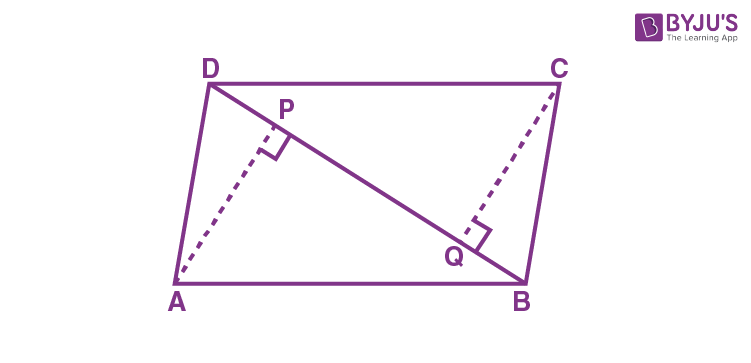Prove that (i) ∆ APB ≅ ∆ CQD (ii) AP = CQ

3. If the diagonals of a parallelogram are equal, prove that it is a rectangle.

Stay tuned with BYJU’S – The Learning App and learn all the class-wise concepts easily by exploring more videos.

## Frequently Asked Questions on Properties of a Parallelogram

### What are the properties of the parallelogram?

The properties of the parallelogram are:
The opposite sides of a parallelogram are parallel and congruent
The consecutive angles of a parallelogram are supplementary
The opposite angles are equal
A diagonal bisect the parallelogram into two congruent triangles
Diagonals bisect each other

### What are the two special types of a parallelogram?

The two special types of a parallelogram are square and rectangle.

### What is the order of rotational symmetry of a parallelogram?

The order of rotational symmetry of a parallelogram is 2.

### Does a parallelogram have reflectional symmetry?

No, a parallelogram does not have reflectional symmetry.